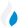•Trade Pi
•Trade
•Exchange
•Trade Pi
•Trade
•Exchange
\$1.09T
Total marketcap
\$64.75B
Total volume
40.85%
BTC dominance
•Trade Pi
•Trade
•Exchange

# near to THB Exchange Rate - 1 NEAR Protocol in THB

77.407333570377
• BTC 0.0001
• ETH 0.0015
Vol [24h]
\$968,212.02

## near to THB converter

Exchange Pair Price 24h volume
Bitkub NEAR/THB \$2.34 \$934.89K
Bitazza NEAR/THB \$2.35 \$25.68K
Zipmex NEAR/THB \$2.31 \$121.4

## NEAR/THB Exchange Rate Overview

Name Ticker Price % 24h 24h high 24h low 24h volume
NEAR Protocol near \$2.34 2.3633% \$2.36 \$2.27 \$156.67M

Selling 1 NEAR Protocol near you get 77.407333570377 THB.

NEAR Protocol Jan 16, 2022 had the highest price, at that time trading at its all-time high of \$20.44.

380 days have passed since then, and now the price is 11.47% of the maximum.

Based on the table data, the NEAR vs THB exchange volume is \$968,212.02.

Using the calculator/converter on this page, you can make the necessary calculations with a pair of NEAR Protocol to THB.

## Q&A

### What is the current NEAR to THB exchange rate?

Right now, the NEAR/THB exchange rate is 77.407333570377.

### What has been the NEAR Protocol to THB trading volume in the last 24 hours?

Relying on the table data, the NEAR Protocol to THB exchange volume is \$968,212.02.

### How can I calculate the amount of THB? / How do I convert my NEAR Protocol to THB?

You can calculate/convert THB from NEAR to THB converter. Also, you can select other currencies from the drop-down list.

## NEAR to THB Сonversion Table

NEAR THB
0.2 NEAR = 15.4 THB
0.4 NEAR = 30.9 THB
0.8 NEAR = 61.9 THB
1 NEAR = 77.4 THB
2 NEAR = 154.8 THB
4 NEAR = 309.6 THB
6 NEAR = 464.4 THB
30 NEAR = 2,322.2 THB
50 NEAR = 3,870.3 THB
500 NEAR = 38,703.6 THB
5000 NEAR = 387,036.6 THB
50000 NEAR = 3,870,366.6 THB
500000 NEAR = 38,703,666.7 THB